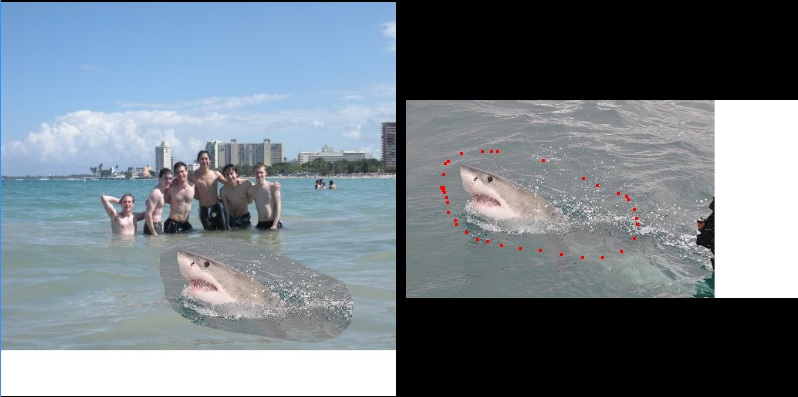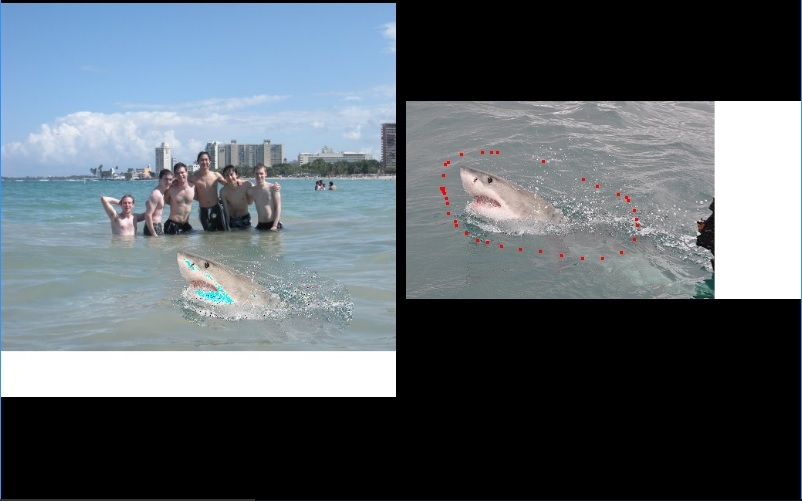# poisson image edittingI knew this algorithms in 2008 which is very simple to implement but I didn’t try it. The day before yesterday I implemented it using OpenGL and SOIL image library. For this simple program ,I don’t want to use the OpenCV. However a bug wasted me a whole day to find the problem.

Suppose we have two variables to sub:

``````unsigned char a=150,b=151;
float f = (float)a-(float)b;
``````

The result is about hundreds,it is very strange. Finally I added these code lines between the above two lines:

``````float af= (float)a;
float bf = (float)b;
float ff = af-bf;
``````

Both f and ff are now correct. When we use VS201x such as VS2015, we sometimes encounter these similar bugs from their products.

The program is tested using the images from internet.

The simple program can be downloaded here (warning : the size of a image should be power of 2. My machine onlye support OpenGL 1 so I didn’t use OpenGL 2 to show images of any size ).

Written on October 16, 2016